# NCERT Solutions For Class 9 Math Chapter – 10 Exercise – 10.6

NCERT Solutions For Class 9 Math Chapter – 10 Exercise – 10.6

Q1.  Prove that the line of centers of two intersecting circles subtends equal angels at wo points of intersection.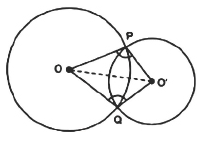Solution:-

∆OAO’ and ∆OBO’

OA = OB (Radii of circle with center O)

O’A = O’B (Radii of circle with center O’)

OO’ = OO’ (Common)

Therefore by SSS criterion ∆OAO’ and ∆OBO’ are congruent to each other.

By CPCT ∟OAO’ = ∟OBO’

Hence it is proved that the line of centers of two intersecting circles subtends equal angels at it the two points of intersection.

Q2. Two chords AB and CD of lengths 5 cm and 11 cm respectively of a circle are parallel to each other and are on opposite of its center If the distance parallel between AB and CD is 6 cm Find the radius of the circle.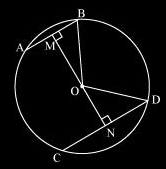Solution:-

AB = 5 cm

CD = 11 cm

MB = 2.5 cm

ND = 5.5 cm

Let OM = x cm and ON = 6 – x cm

∆OMB

By Pythagoras theorem ,

OM2 + MB2 = OB2

X2 + 252 = OB2

X2 + 6.25 = OB2   (I)

∆OND

By Pythagoras theorem

ON2 + ND2 = OD2

• X)2+ 5.52= OD2

36 + x2 – 12x + 30.25 = OD2

X2  – 12x + 66.25 = OD2

OB and OD are the radii of the circle Therefore

OB = OD.

Equation (I) and (ii) we get,

X2 + 6.25 = x2 – 12x + 66.25

12x = 60

X = 5

Substituting  the value of x in (1) or (2)  we get the radius of circle = 5.59 cm.

Q3. The length of two parallel chords of a circle are 6 cm and 8 cm If the smaller chord is at distance 4 cm from the center What is the distance of the other chord from the center?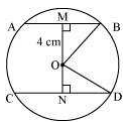Solution:-

AB = 6 cm

CD = 8 cm

MB = 3 cm

ND = 4 cm

Given OM = 4 cm  and let ON = x cm

∆OMB

OM2 + MB2 = OB2

42 + 32 = OB2

OB2 = 25

OB = 5 cm

OB and OD are the radii of the circle. Therefore OD = OB = 5 cm

Consider ∆OND

ON2 + ND2 = OD2

X2 + 42  = 52

X = 3

The distance of the chord CD from the center is 3 cm

Q4. Let  the vertex of an angel ABC be located outside a circle and let the sides of the angel intersect equal chord AD and CE with the circle Prove that ABC is equal to half the difference of the angels subtended b the chords AC and DE at the center.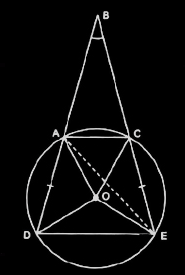Solution:-

∆AOD and ∆COE,

OA = OC

OD = OE

∆AOD  ∆COE (SSS congruence Rule)

∟ODA = ∟OEC [By CPCT]

∟OAD = ∟ODA  (As OA = OD)

∟OAD = ∟OCE = ∟ODA = ∟OEC

Let ∟OAD = ∟OCE = ∟ODA = ∟OEC = X

In ∆OAC ,

OA = OC

∟OCA = ∟OAC

∆ODE,

OD = OE

∟OED = ∟ODE

X + A + X + Y = 1800

2X + A + Y = 1800

Y = 1800 – 2x – a

∟DOE = 1800 – 2Y

∟AOC = 1800 – 2a

∟DOE  – ∟AOC = 2A – 2Y = 2A – 2 (1800  – 2X – A)

= 4a + 4x – 3600

∟BAC + ∟CAD = 1800 (Linear pair)

∟BAC = 1800 – ∟CAD = 1800 – (a+x)

∟ACB = 1800 – (a+x)

In ∆ABC,

∟ABC + ∟BAC + ∟ACB = 1800

∟ABC = 1800 – ∟BAC –  ∟ACB

= 1800 – (1800 – a – x) – (180 – a – x)

= 2a + 2x – 1800

= 1/2[4a + 4x – 3600]

Using Equation (5)

∟ABC = 1/2(∟DOE – ∟AOC)

Q5. Prove that the circle drawn with any side of a rhombus as diameter passes through the point of intersection of it diagonals.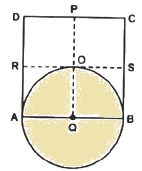Solution:-

∟COD = 900

Also, in rhombus, the diagonal intersect each other at 900.

∟AOB = ∟B0C = ∟COD = ∟DOA = 900

O has to lie on the circle.

Q6.  ABCD is a parallelogram the circle through A , B and C intersect CD at E. Prove that AE = AD.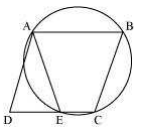Solution:Here, ABCE is a cyclic quadrilateral. In a cyclic quadrilateral, the sum of the opposite angles is 180°.

So, ∠AEC+∠CBA = 180°

As ∠AEC and ∠AED are linear pair,

∠AEC+∠AED = 180°

Or, ∠AED = ∠CBA … (1)

We know in a parallelogram; opposite angles are equal.

So, ∠ADE = ∠CBA … (2)

Now, from equations (1) and (2) we get,

Now, AD and AE are angles opposite to equal sides of a triangle,

7. AC and BD are chords of a circle which bisect each other. Prove that (i) AC and BD are diameters; (ii) ABCD is a rectangle.

Solution: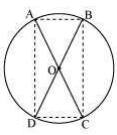Here chords AB and CD intersect each other at O.

Consider ΔAOB and ΔCOD,

∠AOB = ∠COD (They are vertically opposite angles)

OB = OD (Given in the question)

OA = OC (Given in the question)

So, by SAS congruency, ΔAOB ≅ ΔCOD

Also, AB = CD (By CPCT)

Similarly, ΔAOD ≅ ΔCOB

Or, AD = CB (By CPCT)

In quadrilateral ACBD, opposite sides are equal.

So, ACBD is a parallelogram.

We know that opposite angles of a parallelogram are equal.

So, ∠A = ∠C

Also, as ABCD is a cyclic quadrilateral,

∠A+∠C = 180°

⇒∠A+∠A = 180°

Or, ∠A = 90°

As ACBD is a parallelogram and one of its interior angles is 90°, so, it is a rectangle.

∠A is the angle subtended by chord BD. And as ∠A = 90°, therefore, BD should be the diameter of the circle. Similarly, AC is the diameter of the circle.

8. Bisectors of angles A, B and C of a triangle ABC intersect its circumcircle at D, E and F respectively. Prove that the angles of the triangle DEF are 90°–(½)A, 90°–(½)B and 90°–(½)C.

Solution:

Consider the following diagram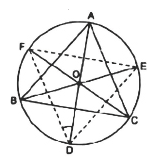Here, ABC is inscribed in a circle with center O and the bisectors of ∠A, ∠B and ∠C intersect the circumcircle at D, E and F respectively.

Now, join DE, EF and FD

As angles in the same segment are equal, so,

∠FDA = ∠FCA ————-(i)

∠FDA = ∠EBA ————-(i)

By adding equations (i) and (ii) we get,

∠FDA+∠EDA = ∠FCA+∠EBA

Or, ∠FDE = ∠FCA+∠EBA = (½)∠C+(½)∠B

We know, ∠A +∠B+∠C = 180°

So, ∠FDE = (½)[∠C+∠B] = (½)[180°-∠A]

∠FDE = [90-(∠A/2)]

In a similar way,

∠FED = [90° -(∠B/2)] °

And,

∠EFD = [90° -(∠C/2)] °

9. Two congruent circles intersect each other at points A and B. Through A any line segment PAQ is drawn so that P, Q lie on the two circles. Prove that BP = BQ.

Solution:

The diagram will be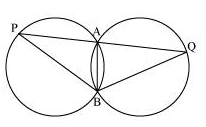Here, ∠APB = ∠AQB (as AB is the common chord in both the congruent circles.)

Now, consider ΔBPQ,

∠APB = ∠AQB

So, the angles opposite to equal sides of a triangle.

∴ BQ = BP

10. In any triangle ABC, if the angle bisector of ∠A and perpendicular bisector of BC intersect, prove that they intersect on the circumcircle of the triangle ABC.

Solution:

Consider this diagram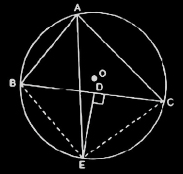Here, join BE and CE.

Now, since AE is the bisector of ∠BAC,

∠BAE = ∠CAE

Also,

∴arc BE = arc EC

This implies, chord BE = chord EC

Now, consider triangles ΔBDE and ΔCDE,

DE = DE     (It is the common side)

BD = CD     (It is given in the question)

So, by SSS congruency, ΔBDE ΔCDE.

Thus, ∴∠BDE = ∠CDE

We know, ∠BDE = ∠CDE = 180°

Or, ∠BDE = ∠CDE = 90°

∴ DE ⊥ BC (hence proved).